###Author Topic: [code snippet] function HTTPEncode  (Read 2765 times)

0 Members and 1 Guest are viewing this topic.

####KeYDoN

• Major
•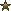• Posts: 60##### [code snippet] function HTTPEncode
« on: April 07, 2007, 08:26:39 am »
Script Name: HTTPEncode
Script Description: This function will encode a string(Source) to be safe for use in a URL.
Original Author(s): KeYDoN
Core Version: 0.5.0

Sick of waiting for 2.6 or some surprising 2.5.5 version?
here you go:

Code:
Code: [Select]
`function HTTPEncode(Str: String):String;vari: integer;s: string;begin for i:=1 to length(Str) do begin s:= Copy(Str,i,1); if s=' ' then result:=result+'%20' else if s='!' then result:=result+'%21' else if s='"' then result:=result+'%22' else if s='#' then result:=result+'%23' else if s='\$' then result:=result+'%24' else if s='%' then result:=result+'%25' else if s='&' then result:=result+'%26' else if s='''' then result:=result+'%27' else if s='(' then result:=result+'%28' else if s=')' then result:=result+'%29' else if s='*' then result:=result+'%2A' else if s='+' then result:=result+'%2B' else if s=',' then result:=result+'%2C' else if s='-' then result:=result+'%2D' else if s='.' then result:=result+'%2E' else if s='/' then result:=result+'%2F' else if s=':' then result:=result+'%3A' else if s=';' then result:=result+'%3B' else if s='<' then result:=result+'%3C' else if s='=' then result:=result+'%3D' else if s='>' then result:=result+'%3E' else if s='?' then result:=result+'%3F' else if s='@' then result:=result+'%40' else if s='[' then result:=result+'%5B' else if s='\' then result:=result+'%5C' else if s=']' then result:=result+'%5D' else if s='^' then result:=result+'%5E' else if s='_' then result:=result+'%5F' else if s='`' then result:=result+'%60' else if s='{' then result:=result+'%7B' else if s='|' then result:=result+'%7C' else if s='}' then result:=result+'%7D' else if s='~' then result:=result+'%7E' else if s='§' then result:=result+'%A7' else if s='²' then result:=result+'%B2' else if s='³' then result:=result+'%B3' else if s='´' then result:=result+'%B4' else if s='µ' then result:=result+'%B5' else result:=result+s; end;end;`
If i forgot something, tell me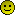« Last Edit: April 07, 2007, 08:36:23 am by KeYDoN »

####truup

• Soldier
•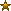• Posts: 243##### Re: [code snippet] function HTTPEncode
« Reply #1 on: April 07, 2007, 08:38:04 am »
If i forgot something, tell meUmm... Spacebar? :O

####KeYDoN

• Major
•• Posts: 60##### Re: [code snippet] function HTTPEncode
« Reply #2 on: April 07, 2007, 08:42:30 am »
is on the first position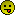####xmRipper

• Soldat Beta Team
• Flagrunner
•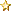•• Posts: 742##### Re: [code snippet] function HTTPEncode
« Reply #3 on: April 07, 2007, 08:54:33 am »
Umm... Spacebar? :O

Quote
if s=' ' then result:=result+'%20'
CoFounder / CTO @ Macellan
Founder Turkish Soldat Community

####truup

• Soldier
•• Posts: 243##### Re: [code snippet] function HTTPEncode
« Reply #4 on: April 07, 2007, 10:31:16 am »

####Riax##### Re: [code snippet] function HTTPEncode
« Reply #5 on: April 07, 2007, 07:19:28 pm »
I think you should remove the period, colon, and forward slash from the function; the period and slash are common to all URI's, and the colon is commonly used to designate a port after the hostname in a URL.

Also, the question mark, equals sign, and ampersand are used in the URL's of most sites which utilize some form of server-side scripting, such as PHP or ASP, to generate dynamic content.

Other vaild URI characters found in the function are as follows:

#
+
- (hyphen)
_ (underscore)
~

There may be others, but those are the ones that caught my eye.
« Last Edit: April 07, 2007, 07:24:10 pm by Riax »Founder, Simplexity Network // Fan, Machine Supremacy

####KeYDoN

• Major
•• Posts: 60##### Re: [code snippet] function HTTPEncode
« Reply #6 on: April 07, 2007, 07:24:22 pm »
You cannot exclude these signs.

You may not encode the whole URL, just the parameters!
http://enesce.com/help/html/Functions/HTTPEncode.html

####Riax##### Re: [code snippet] function HTTPEncode
« Reply #7 on: April 07, 2007, 07:31:51 pm »
I stand thoroughly corrected. Serves me right for not double-checking the scripting manual to make sure I knew exactly what the function will do in v2.6.